Exponents

Chapter 13 Class 7 Exponents and Powers
Concept wise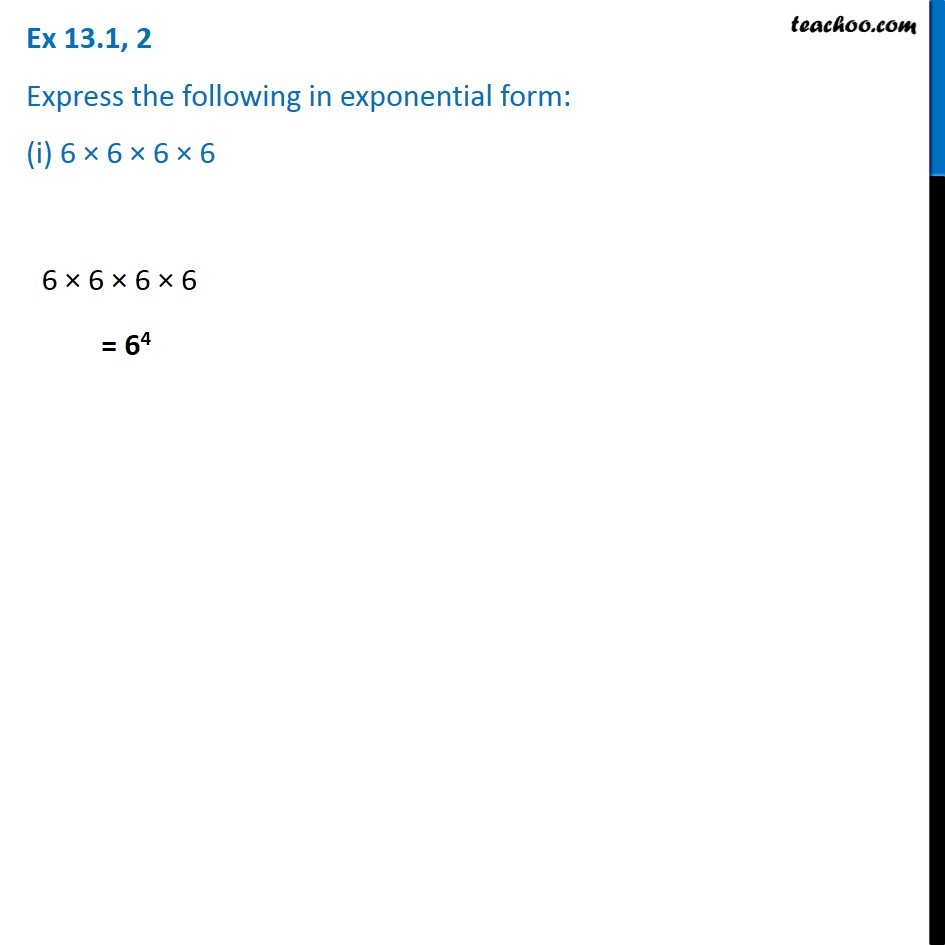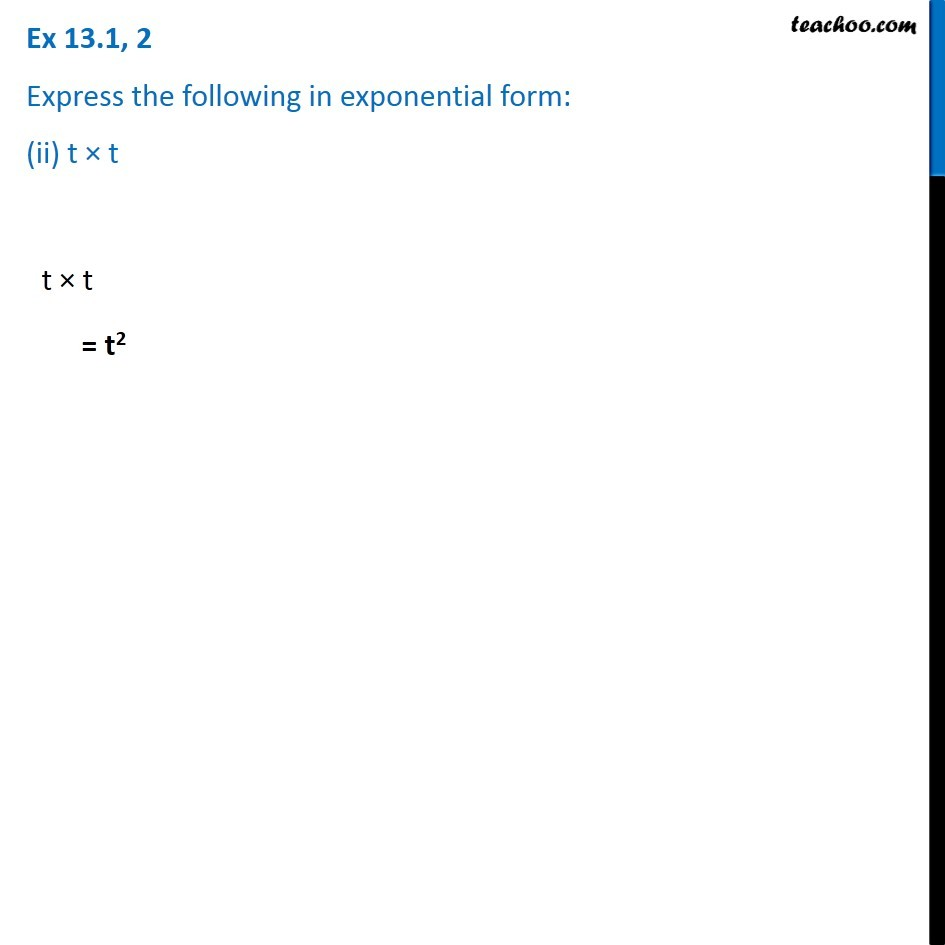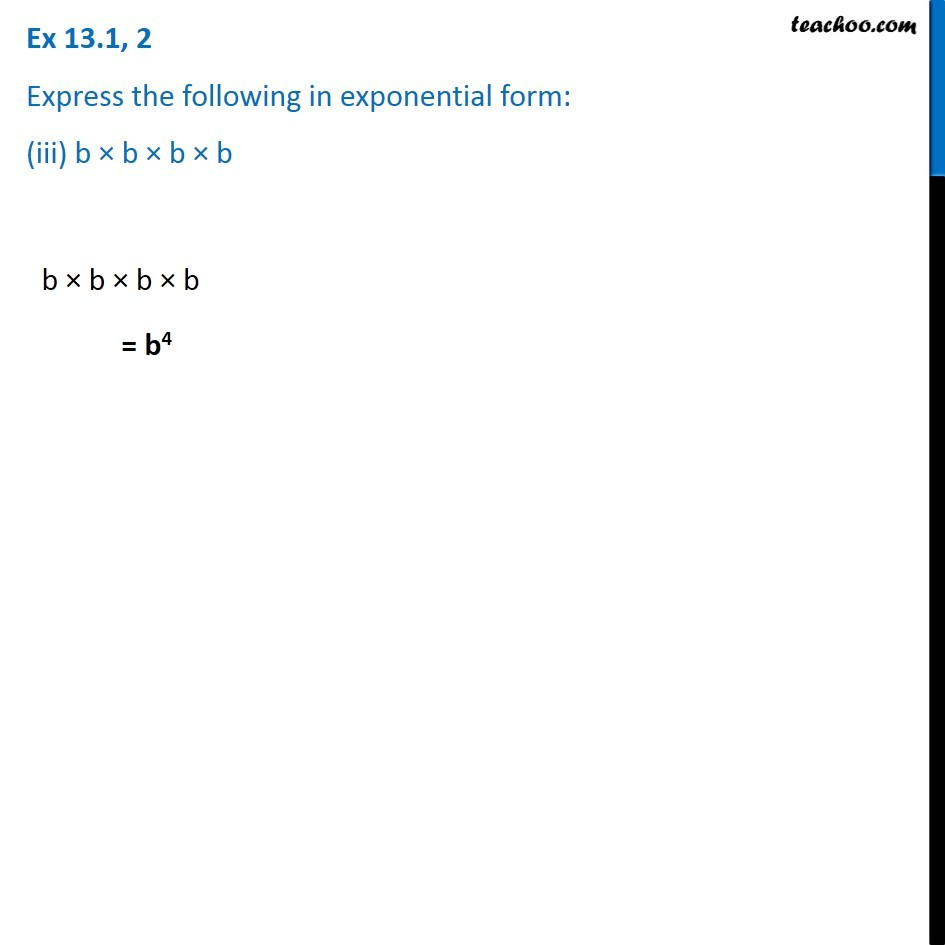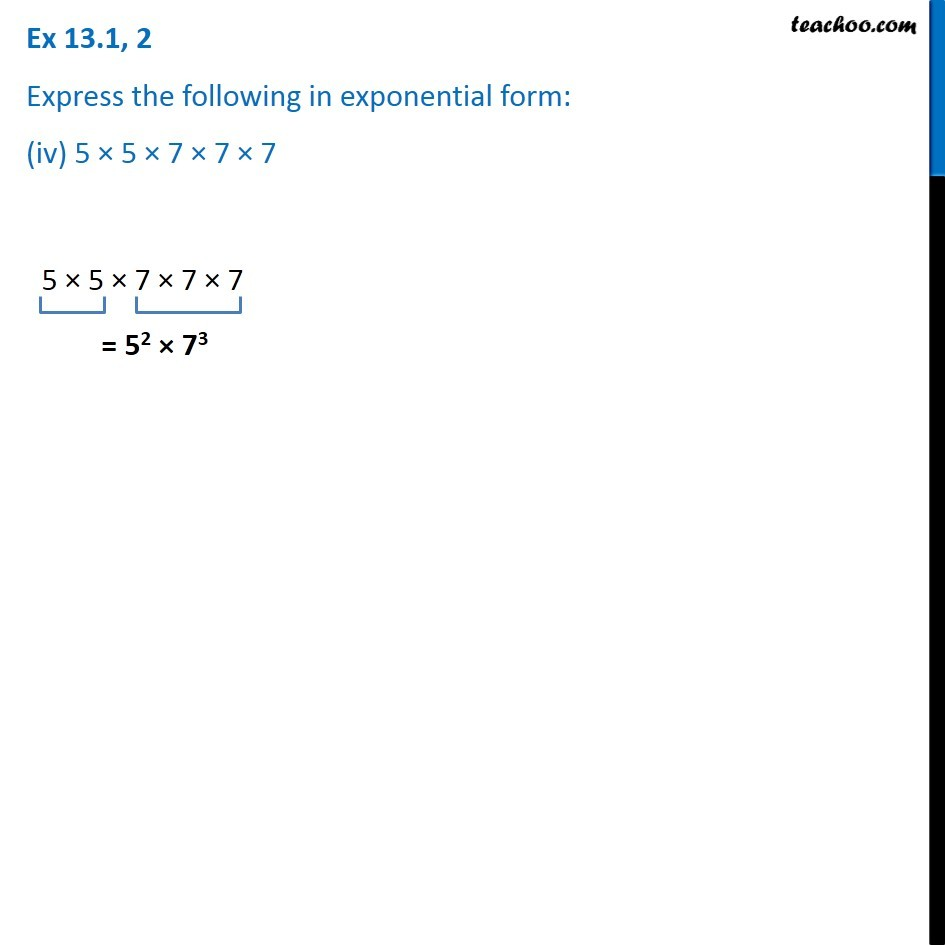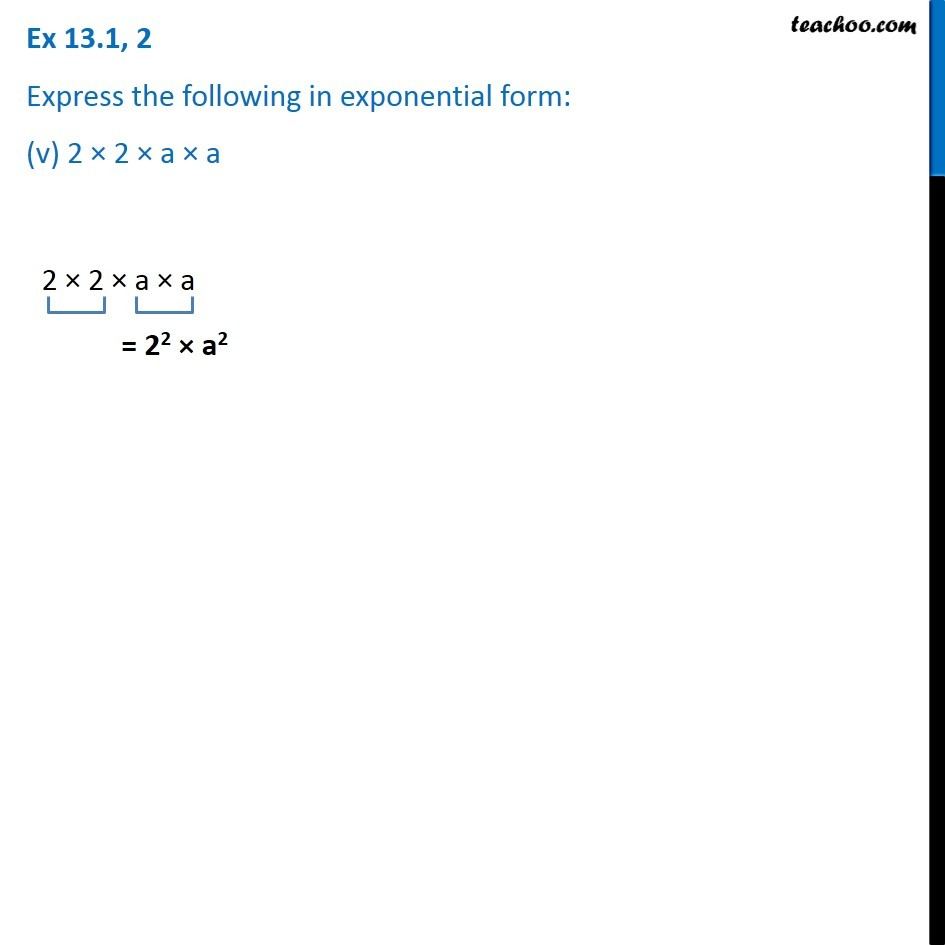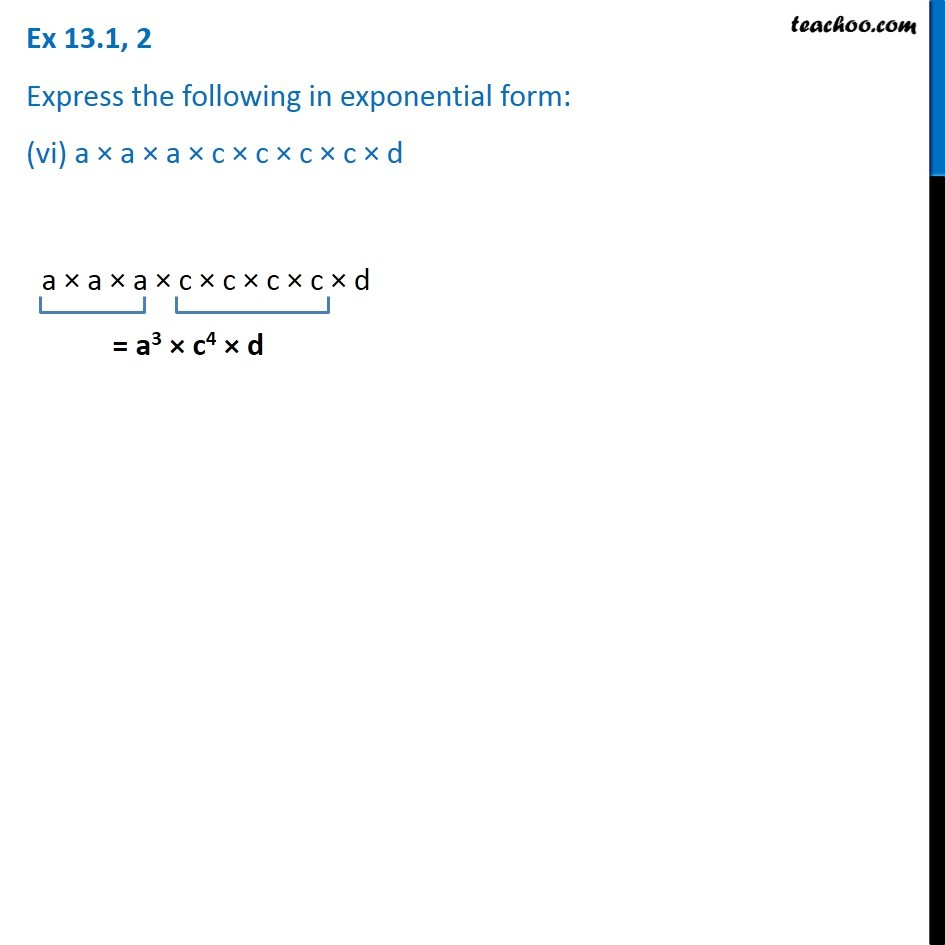Introducing your new favourite teacher - Teachoo Black, at only ₹83 per month

### Transcript

Ex 13.1, 2 Express the following in exponential form: (i) 6 × 6 × 6 × 6 6 × 6 × 6 × 6 = 64 Ex 13.1, 2 Express the following in exponential form: (ii) t × t t × t = t2 Ex 13.1, 2 Express the following in exponential form: (iii) b × b × b × b b × b × b × b = b4 Ex 13.1, 2 Express the following in exponential form: (iv) 5 × 5 × 7 × 7 × 7 5 × 5 × 7 × 7 × 7 = 52 × 73 Ex 13.1, 2 Express the following in exponential form: (v) 2 × 2 × a × a 2 × 2 × a × a = 22 × a2 Ex 13.1, 2 Express the following in exponential form: (vi) a × a × a × c × c × c × c × d a × a × a × c × c × c × c × d = a3 × c4 × d